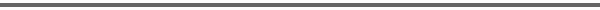Journal Home Page Cumulative Index List of all Volumes Complete Contentsof this Volume Previous Article Next Article Journal of Convex Analysis 10 (2003), No. 2, 325--350 Copyright Heldermann Verlag 2003Multiscale Relaxation of Convex Functionals Irene Fonseca Dept. of Mathematical Sciences, Carnegie Mellon University, Pittsburgh, PA 15213, U.S.A., fonseca@andrew.cmu.edu Elvira Zappale Dip. di Ingegneria dell'Informazione e Matematica Applicata, Universitŕ degli Studi di Salerno, 84084 Fisciano, Italy, zappale@diima.unisa.it[Abstract-pdf] The $\Gamma$-limit of a family of functionals $$u\mapsto \int_{\Omega}f\left(\frac{x}{\e},\frac{x}{\e^2},D^su\right)\, dx$$ is obtained for $s=1,2$ and when the integrand $f=f(x,y,v)$ is a continuous function, periodic in $x$ and $y$, and convex with respect to $v$. The $3$-scale limits of second order derivatives are characterized. Keywords: Convexity, periodicity, multiscale limits, Γ-convergence, A-quasiconvexity, measurability selection criterion. MSC 2000: 35G99, 49J40, 49J45, 74G65. FullText-pdf (398 K)